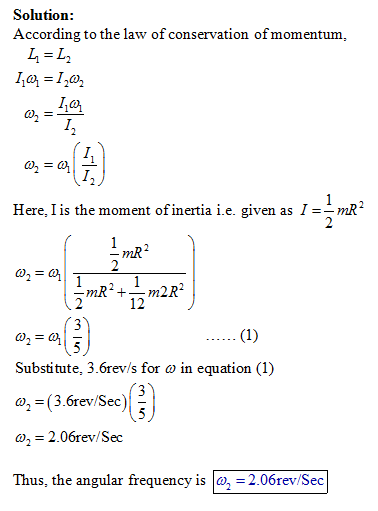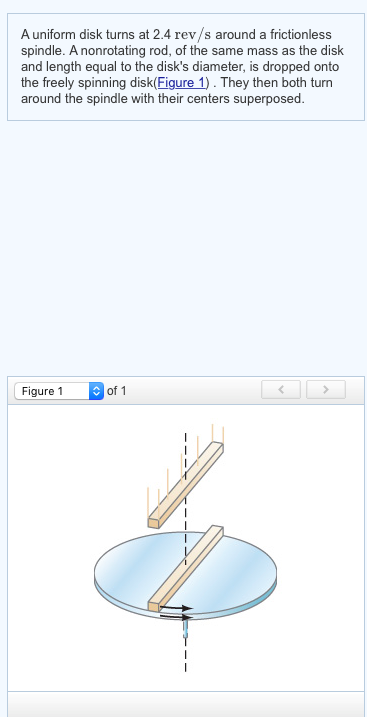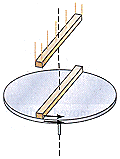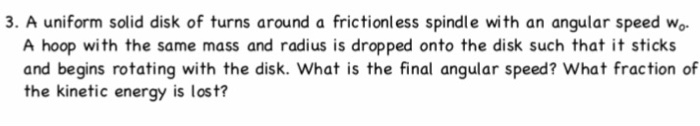# A uniform disk turns at 3.6 rev/s around a frictionless spindle. A non rotating rod, of the same mass as the disk and l...

A uniform disk turns at 3.6 rev/s around a frictionless spindle. A non rotating rod, of the same mass as the disk and length equal to the disk's diameter, is dropped onto the freely spinning disk . They then both turn around the spindle with their centers superposed.

What is the angular frequency in rev/s of the combination?##### Add Answer of: A uniform disk turns at 3.6 rev/s around a frictionless spindle. A non rotating rod, of the same mass as the disk and l...
Similar Homework Help Questions
• ### A uniform disk turns at 3.7 rev/s around a frictionless spindle. A nonrotating rod, of the same mass as the disk and len...

A uniform disk turns at 3.7 rev/s around a frictionless spindle. A nonrotating rod, of the same mass as the disk and length equal to the disk's diameter, is dropped onto the freely spinning disk. They then turn together around the spindle with their centers superposed. What is the angular frequency in rev/s of the combination?

• ### A uniform disk turns at 3.6 rev/s around a frictionless central axis. A nonrotating rod, of the same mass as the dis...

A uniform disk turns at 3.6 rev/s around a frictionless central axis. A nonrotating rod, of the same mass as the disk and length equal to the disk's diameter, is dropped onto the freely spinning disk(Figure 1). They then turn around the spindle with their centers superposed. Figure 1 of 1 Part A What is the angular frequency in rev/s of the combination? # - - - -

• ### A uniform disk turns at 2.7 rev/s around a frictionless central axis. A nonrotating rod, of the same mass as the disk a...

A uniform disk turns at 2.7 rev/s around a frictionless central axis. A nonrotating rod, of the same mass as the disk and length equal to the disk's diameter, is dropped onto the freely spinning disk(Figure 1) . They then turn around the spindle with their centers superposed. What is the angular frequency in rev/s of the combination?

• ### What is the angular frequency in rev/s of the combination? A uniform disk turns at 2.4 rev/s around a frictionless sp...What is the angular frequency in rev/s of the combination? A uniform disk turns at 2.4 rev/s around a frictionless spindle. A nonrotating rod, of the same mass as the disk and length equal to the disk's diameter, is dropped onto the freely spinning disk(Figure 1). They then both turn around the spindle with their centers superposed. Figure 1 of 1

• ### Angular Frequency problem

A uniform disk turns at 3.7 rev/s around a frictionless spindle. A nonrotating rod, of the same mass as the disk and length equal to the disk's diameter, is droppedonto the freely spinning disk. They then turn together around the spindle with their centers superposed. What is the angular frequency in rev/s of the combination?

• ### angular frequencyA uniform disk turnsat 3.6 around a frictionless spindle. A nonrotating rod, of thesame mass as the disk and length equal to the disk's diameter, isdropped onto the freely spinning disk.They then turn togetheraround the spindle with their centers superposed.(Intro 1 figure)What is the angular frequency in of the combination?(Please explain well, thanks)

• ### physics

A uniform disk turns at 1.8 around a frictionless spindle. A nonrotating rod, of the same mass as the disk and length equal to the disk's diameter, is dropped onto thefreely spinning disk . They then both turn around the spindle with their centers superposed

• ### Rotational MotionA uniform disk turns at 9.5 rev/saround a frictionless spindle. A nonrotating rod, of the same massas the disk and length equal to the disk's diameter, is droppedontothe freely spinning disk, see the figure. They then turntogether around the spindle with their centers superposed. What isthe angular velocity of thecombination? rev/sHelp!

• ### 3. A uniform solid disk of turns around a frictionless spindle with an angular speed wo...3. A uniform solid disk of turns around a frictionless spindle with an angular speed wo A hoop with the same mass and radius is dropped onto the disk such that it sticks and begins rotating with the disk. What is the final angular speed? What fraction of the kinetic energy is lost?